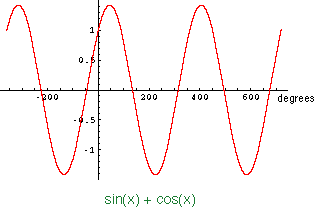Quandaries and Queries WHAT IS THE PERIOD OF SIN(X)+COS(X)? PLEASE EXPLAIN WITH A GRAPH. Hi, The periods of sin(x) and cos(x) are both 360o so sin(x) + cos(x) repeats on any interval of length 360o. Thus 360o is a period of sin(x) + cos(x). The question is then, is 360o the period of sin + cos(x)? That is, is 360o the length of the shortest interval on which sin + cos(x) repeats? This is where a graph is useful. I plotted sin(x) + cos(x) for x from -360o to 720oFrom the graph you can see that there is a repeat on intervals of length 360o but no shorter interval where the graph repeats. Thus the period of sin + cos(x) is 360o. Penny Go to Math Central